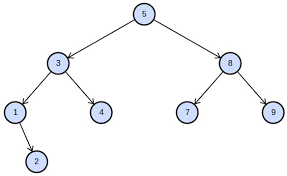# #351. 新年的叶子### 样例一

#### input

3
1 2
2 3



#### output

1



### 样例二

#### input

6
1 2
2 3
1 4
4 5
1 6



#### output

499122178



### 限制与约定

1 12 $\le 10$
2 18 $\le 3\times 10^3$ $1$
3 20 $\le 5\times 10^5$ 树上任意两点距离（距离定义为两点间最短路边的条数）$\le 3$
4 15 $\le 5\times 10^5$ 任意点度数$\le 3$
5 35 $\le 5\times 10^5$ $1,2,3,4$